The work was published in: Mat. Zametki 60 (1996), no. 3, pp. 333-355;
English translation: Math. Notes. 60 (1996), no. 3, pp. 248-263.

S U M M A R Y

41A17 41A44

B a b e n k o A. G.
Exact Jackson-Stechkin type inequality in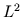space of functions on the multidimensional sphere.
In this paper we prove Jackson-Stechkin type inequality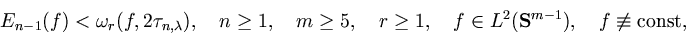which is exact for every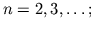here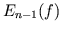is the best approximation of the function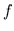by spherical polynomials of degree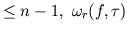is the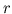th modulus of continuity ofbased on translation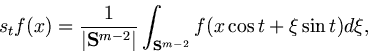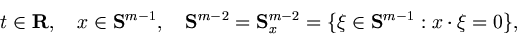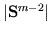is the measure of the unit Euclidean sphere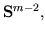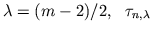is the first positive zero of the Gegenbauer's cosine polynomial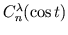.